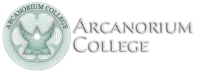## Arcanorium CollegeCollege News and Views

Thursday, 01 December 2022 18:18

## The Anti-Singularity Theorems Featured

THE ANTI-SINGLARITY THEOREMS.

We must remain highly suspicious of any theory which asserts that an infinite amount of any physical quantity exists. We can never observe an infinite quantity of anything, and we have no reason to suppose that an infinite quantity of anything can exist. The concept only arises when we introduce it by mistakenly assuming that something else has no limits or by erroneously dividing something by nothing.

If spacetime and mass/energy have a mutual dependency, then we have no reason to assume that spacetime exists independently and infinitely beyond the presence of mass/energy.

Contemporary theoretical physics suffers from the delusion that mass/energy can become concentrated in an arbitrarily small volume, including zero volume. This has led to the assumption that black holes must contain singularities and that this universe arose from a zero dimensional singularity and the assumption that fundamental quanta can exist as zero dimensional point particles.

The Schwarzschild  Solution of General Relativity: -

r = 2Gm/c^2 shows that an event horizon at radius r will arise when a given mass m collapses to that radius under its own gravity creating a black hole.

The Penrose-Hawking Singularity Theorems assert that nothing can prevent further collapse and thus that a spacetime singularity consisting of a zero-dimensional point of infinite density must inevitably form within any black hole.

The Godel Solution of General Relativity: -

w = 2sqrt(piGp) does however indicate that something will prevent the collapse of matter into spacetime singularities.

‘Matter everywhere rotates relative to the compass of inertia with an angular velocity (w) of twice the square root of pi times the gravitational constant (G) times the density (p)’.

As matter becomes denser, spacetime curvature increases and a compact body acquires a radius excess which gives it more volume on the inside than an outside observer would expect from its exterior radius by assuming 4/3 pi r^3 for flat Euclidian space.

When a body contracts to r = 2Gm/pi c^2  (or pi r = 2Gm/c^2) the radius excess makes its internal ‘diameter’ equal to half of its circumference and the body becomes a Glome Hypersphere. With a volume of 2pi^2 r^3 or 2L^3/pi where L means antipode distance.

The Godel Metric w = 2sqrt(piGp) then supplies the internal metric of such hyperspheres in the reference frame of internal observers, and it shows that such bodies must have an angular velocity w.

Substituting hypersphere volume into the Godel metric shows that a Glome hypersphere will rotate with an angular velocity of pi c/L and hence a frequency f of c/2L and have balanced centripetal and centrifugal accelerations a of c^2/L.

Given that: -    w = 2sqrt(piGp)w = 2pi f,   p = mass m/volume v,    2Gm/L = c^2

And that hypersphere volume v = 2L^3/pi

Then: -    w = 2sqrt(piGp),         w^2 = 4pi^2Gm/2L^3,            w^2 = pi^2c^2/L^2,

w = pi c/L,        2pi f = pi c/L,      f = c/2L,    a = c^2/L.

The mass inside a Glome hypersphere does not rotate as a simple sphere with a single axis of rotation, but rather it rotates about the randomly orientated axes of the Hopf Fibration.

Hence such Glome Hyperspheres cannot contract any further because that would cause them to rotate faster than light. This falsifies the Penrose-Hawking Singularity Theorems for both black holes and the universe as a whole.

If the mass of the universe remains constant, then so does its size, despite appearances. (See the Hypersphere Cosmology redshift-distance and hyperspherical lensing relationships.)

Now if such hypersphere mechanics forbids the formation of spacetime singularities at cosmic and astronomical scales then it most likely also forbids the formation of fundamental zero dimensional point particles. Yet we cannot usefully regard quanta as miniature cannon balls.

Quanta also have characteristic wavelengths many orders of magnitude greater than the antipode lengths suggested by L = 2Gm/c^2 for such particles. Yet the wavelengths of quanta have a directional quality which we most commonly observe in the direction of propagation rather than transverse to it.

The following calculation shows that given the Planck-Einstein relationship E = hf and the Godel metric w = 2sqrt(piGp), quanta with mass have a definite volume: -

Given that: -  E = hf,    w = 2sqrt(piGd),   w = 2pi f,   E = mc^2,   p = mass m/volume v

Then: -      f = mc^2/h,         w = 2pi mc^2/h,         2pi mc^2/h = 2sqrt(piGm/v),

pi^2 m^2c^4/h^2 = piGm/v,       pi mc^4/h^2 = G/v,

v = Gh^2/pi mc^4

This closely matches the Einstein-Cartan volume of a quantum ~ Gh^2/mc^4  (derived by other means).

Thus, it seems probable that quanta embody a hyper-geometrical component in their probably variable dynamic geometry, rather than existing as simple 3-sphere Glome Hyperspheres. Rotating Hypertoruses of some kind may supply a solution. Research continues.

As quanta have a spatial volume, then that volume sets yet another limit to the compressibility of matter and its maximum possible density. This also sets a minimum bound for the mass of any black hole.Arcanorium College Interroga Omnia – Question all things. Arcanorium College consists of a Natural Philosophy Faculty with two Departments: - The Department